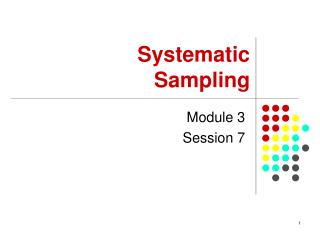# Module 3 Session 7 - PowerPoint PPT PresentationDownload PresentationModule 3 Session 7

Module 3 Session 7Download Presentation## Module 3 Session 7

- - - - - - - - - - - - - - - - - - - - - - - - - - - E N D - - - - - - - - - - - - - - - - - - - - - - - - - - -
##### Presentation Transcript

1. Systematic Sampling Module 3 Session 7

2. Session Objectives • To introduce basic sampling concepts in systematic sampling • Demonstrate how to select a random sample using systematic sampling design

3. Sample Selection Procedure • List all the units in the population from 1,2,…,N – Sampling frame • Select a random number g in the interval • 1 g K, using a random mechanism e.g. random number tables, where K = • K is called the Sampling Interval • N is the population size; n is the sample size • The random number g is called the random start and constitutes the first unit of the sample

4. Sample Selection Procedure • Take every kth unit after the random start • The selected units will be • g, g+k, g+2k, g+3k, g+4k, …,g+(n-1)k • Until we have n units • Example N =10000, n=100 • k = =100 • Suppose g=87

5. Sample Selection Procedure • We select the following units • 87, 187, 287, 387,…, 9987 • NB: This procedure is however only valid if k is an integer (whole number) • If k is not an integer (whole number) there are a number of methods we can use. We will consider just two of them

6. Sample Selection Procedure • Method 1: Use Circular Sampling • Treat the list as circular so that the last unit is followed by the first • Select a random start g between 1 and N, using a random mechanism • Add the intervals k until n units are selected • Any convenient interval k will result into a random sample

7. Sample Selection Procedure • One suitable suggestion is to choose the integer k closest to the ratio • Method 2: Use Fractional Intervals • Suppose we want to select a sample of 100 units from a population of 21,156. • Calculate k = =211.56 • Select a random start g between 1 and 21156 using a random mechanism

8. Sample Selection Procedure • Suppose g = 582 • Add the interval 21156 successively obtaining exactly 100 numbers • The numbers will be 582, 21738, 42894, … • Divide each number by 100 and round to the nearest whole number to get the selected sample, i.e. • 6, 217, 429, etc

9. Advantages and Disadvantages of Systematic sampling • Advantages: • The major advantage is that it is easy, almost foolproof and flexible to implement • It is especially easy to give instructions to fieldworkers • If we order our list prior to taking the sample, the sample will reflect the ordering and as such can easily give a proportionate sample

10. Advantages and Disadvantages of Systematic sampling • Disadvantages: • The main disadvantage is that if there is an ordering (monotonic trend or periodicity) in the list which is unknown to the researcher, this may bias the resulting estimates • There is a problem of estimating variance from systematic sampling- variance is biased